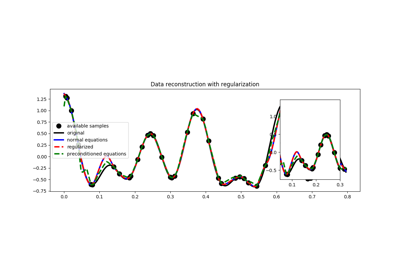# pylops.optimization.sparsity.SPGL1¶

pylops.optimization.sparsity.SPGL1(Op, data, SOp=None, tau=0, sigma=0, x0=None, **kwargs_spgl1)[source]

Solve a constrained system of equations given the operator Op and a sparsyfing transform SOp aiming to retrive a model that is sparse in the sparsyfing domain.

This is a simple wrapper to spgl1.spgl1 which is a porting of the well-known SPGL1 MATLAB solver into Python. In order to be able to use this solver you need to have installed the spgl1 library.

Parameters: Op : pylops.LinearOperator Operator to invert data : numpy.ndarray Data SOp : pylops.LinearOperator Sparsyfing transform tau : float Non-negative LASSO scalar. If different from 0, SPGL1 will solve LASSO problem sigma : list BPDN scalar. If different from 0, SPGL1 will solve BPDN problem x0 : numpy.ndarray Initial guess **kwargs_spgl1 Arbitrary keyword arguments for spgl1.spgl1 solver xinv : numpy.ndarray Inverted model in original domain. pinv : numpy.ndarray Inverted model in sparse domain. info : dict Dictionary with the following information: tau, final value of tau (see sigma above) rnorm, two-norm of the optimal residual rgap, relative duality gap (an optimality measure) gnorm, Lagrange multiplier of (LASSO) stat, 1: found a BPDN solution, 2: found a BP solution; exit based on small gradient, 3: found a BP solution; exit based on small residual, 4: found a LASSO solution, 5: error, too many iterations, 6: error, linesearch failed, 7: error, found suboptimal BP solution, 8: error, too many matrix-vector products. niters, number of iterations nProdA, number of multiplications with A nProdAt, number of multiplications with A’ n_newton, number of Newton steps time_project, projection time (seconds) time_matprod, matrix-vector multiplications time (seconds) time_total, total solution time (seconds) niters_lsqr, number of lsqr iterations (if subspace_min=True) xnorm1, L1-norm model solution history through iterations rnorm2, L2-norm residual history through iterations lambdaa, Lagrange multiplier history through iterations ModuleNotFoundError If the spgl1 library is not installed

Notes

Solve different variations of sparsity-promoting inverse problem by imposing sparsity in the retrieved model .

The first problem is called basis pursuit denoise (BPDN) and its cost function is

$||\mathbf{x}||_1 \quad subj. to \quad ||\mathbf{Op}\mathbf{S}^H\mathbf{x}-\mathbf{b}||_2 <= \sigma,$

while the second problem is the l1-regularized least-squares or LASSO problem and its cost function is

$||\mathbf{Op}\mathbf{S}^H\mathbf{x}-\mathbf{b}||_2 \quad subj. to \quad ||\mathbf{x}||_1 <= \tau$
  van den Berg E., Friedlander M.P., “Probing the Pareto frontier for basis pursuit solutions”, SIAM J. on Scientific Computing, vol. 31(2), pp. 890-912. 2008.

## Examples using pylops.optimization.sparsity.SPGL1¶03. Solvers